# Go Math Grade 3 Answer Key Chapter 7 Division Facts and Strategies Assessment Test

This chapter can improve student’s math skills, by referring to the Go Math Grade 3 Answer Key Chapter 7 Division Facts and Strategies Assessment Test, and with the help of this Go Math Grade 3 Assessment Test Answer Key, students can score good marks in the examination.

Go Math Grade 3 Answer Key Chapter 7 contains all the topics of chapter 7 which helps to test the student’s knowledge. Through this assessment test, students can check their knowledge. This assessment test is also helpful for the teachers to know how much a student understood the topics.

Chapter 7: Division Facts and Strategies Assessment Test

### Test – Page 1 – Page No. 71

Question 1.
Shang shared 28 postcards among 7 different people. Each person received the same number of postcards. How many postcards did Shang give to each person?
28 ÷ 7 = n
7 × n = 28
Options:
a. 4
b. 5
c. 6
d. 21

Explanation:
As Shang shared 28 postcards to 7 different people so 28 ÷ 7 = 4 , 7 × 4 = 28. Shang gave 4 postcards to each person.

Question 2.
Lionel has 14 mittens.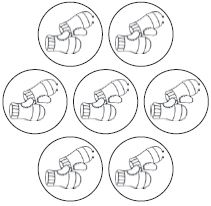Select one number from each column to show the division equation represented by the picture.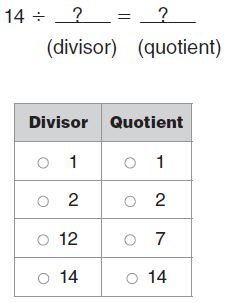14 ÷ ______ = ______

Answer: 14 ÷ 2 = 7.

Explanation: 14 ÷ 2 = 7 which is 7 pairs of mitten given the model.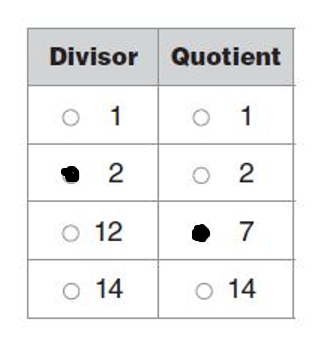### Test – Page 2 – Page No. 72

Question 3.
Fifteen people are going rafting. They brought 5 rafts. An equal number of people ride in each raft. How many people will be in each raft?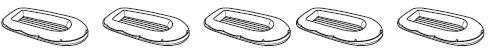______ people

Explanation:
No of people going for rafting = 15
Total no of rafts available = 5
No of people will be in each raft = 15 ÷ 5 = 3 people.

Question 4.
Circle a number for the unknown factor and quotient that makes the equation true.
4 ×= 24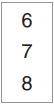= 24 ÷ 4
4 × ______ = 24 ;               ______ = 24 ÷ 4

4×6= 24
6= 24 ÷ 4

Explanation: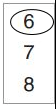= 24= 24 ÷ 4

Question 5.
There are 20 students in science class. There are 10 students sitting at each table. How many tables are there?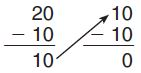Write a division equation to represent the repeated subtraction.
______ ÷ ______ = ______

Answer: 20 ÷ 10 = 2.

Explanation:
Total no of students in class = 20
No of students sitting at each table = 10
Therefore no of tables = 20 ÷ 10 = 2

Question 6.
Complete the chart to show the quotients.63÷9= 7.
72÷9= 8.
81÷9= 9.
90÷9= 10.

Explanation:### Test – Page 3 – Page No. 73

Question 7.
For numbers 7a–7e, select True or False for each equation.
a. 0 ÷ 6 = 6
i. True
ii. False

Explanation: 0 ÷ 6 = 0, so the answer is false.

Question 7.
b. 6 ÷ 6 = 1
i. True
ii. False

Explanation: As 6 ÷ 6 = 1, so the answer is true.

Question 7.
c. 18 ÷ 6 = 2
i. True
ii. False

Explanation: As 18 ÷ 6 = 3, so the answer is false.

Question 7.
d. 54 ÷ 6 = 9
i. True
ii. False

Explanation: As 54 ÷ 6 = 9, so the answer is true.

Question 7.
e. 60 ÷ 10 = 6
i. True
ii. False

Explanation: As 60 ÷ 10 = 6, so the answer is true.

Question 8.
Kaitlyn says that 8 ÷ 2 × 4 is the same as 4 × 2 ÷ 8.
Is Kaitlyn correct or incorrect? Explain.

Answer: No. 8 ÷ 2 × 4 and 4 × 2 ÷ 8 are not the same.

Expplanation:Lets calculate 8 ÷ 2 × 4 = 4 × 4 = 16 by using BODMAS, first we did division later multiplication
4 × 2 ÷ 8 = 4 × ¼ = 1. Thus both results are not same.

Question 9.
Brian is dividing 64 baseball cards equally among 8 friends. How many baseball cards will each friend get?
_______ baseball cards

Explanation:
Total cards available = 64
No of friends = 8
No of baseball cards each gets = 64 ÷ 8 = 8

Question 10.
Tara made $18 selling cookies. She wants to know how many cookies she sold. Tara used this number line.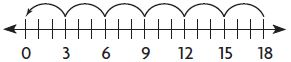Write the division equation that the number line represents. ______ ÷ ______ = ______ Answer: 18 ÷ 3 = 6. Explanation: Possible answers could be if she sells each cookie at$ 3 then 18 ÷ 3 = 6 were sold

### Test – Page 4 – Page No. 74

Question 11.
Each team at a science competition has 6 players. How many teams are there if 42 players are at the competition? Explain the strategy you used to solve the problem.
_____ teams

Explanation:
Given that there is a total of 42 players
Each team has 6 players, therefore the total number of teams = 42 ÷ 6 = 7 teams.

Question 12.
Carly bought 3 packs of baseball cards. Each pack had the same number of cards. She gave 5 cards to her sister. Now she has 19 cards. How many baseball cards were in each pack? Explain how you solved the problem.
_____ baseball cards

Answer: 24 ÷ 3 = 8 baseball cards.

Explanation:
Total packs bought were = 3
No of cards she has = 19
No of cards given to her sister = 5
Total number cards = 19+5 = 24
No of cards in each pack = 24 ÷ 3 = 8 cards.

Question 13.
Andrea used 35 craft sticks to make 7 door hangers. She used the same number of craft sticks for each door hanger. How many craft sticks did Andrea use for each door hanger?
_____ craft stick

Explanation:
Total no of craft sticks used by Andrea = 35
No of door hangers made = 7
Therefore no of craft sticks used for each door hanger = (Total no of craft sticks ÷  no of door hangers)
= 35 ÷ 7
= 5 craft sticks.

Question 14.
For numbers 14a–14e, use the order of operations. Select True or False for each equation.
a. 45 ÷ 5 − 3 = 6
i. True
ii. False

Explanation:
By BODMAS rule
45 ÷ 5 − 3
= 9 – 3
= 6

Question 14.
b. 12 + 4 ÷ 4 = 13
i. True
ii. False

Explanation:
By BODMAS rule
12 + 4 ÷ 4
= 12 + 1
= 13

Question 14.
c. 3 + 7 × 8 = 80
i. True
ii. False

Explanation:
By BODMAS rule
3 + 7 × 8
= 3 + 56
= 59

Question 14.
d. 32 ÷ 8 × 2 = 2
i. True
ii. False

Explanation:
By BODMAS rule
32 ÷ 8 × 2
= 4 × 2
= 8.

Question 14.
e. 40 − 10 × 3 = 10
i. True
ii. False

Explanation:
By BODMAS rule
40 − 10 × 3
= 40 – 30
= 10.

### Test – Page 5 – Page No. 75

Question 15.
Patrick sells homemade pretzels in bags with 9 pretzels in each bag. He sells 54 pretzels in all. How many bags of pretzels does he sell?
______ bags

Explanation:
No of bags sold = (Total no of pretzels / no of pretzels in each bag)
= 54/9
= 6 bags.

Question 16.
Enrique started a table showing a division pattern.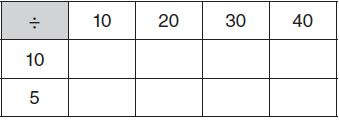Part A
Complete the table.
Compare the quotients when dividing by 10 and when dividing by 5. Describe a pattern you see in the quotients.

10÷10= 1
10÷5= 2
20÷10= 2
20÷5= 4
30÷10= 3
30÷5= 6
40÷10= 4
40÷5= 8.

Explanation: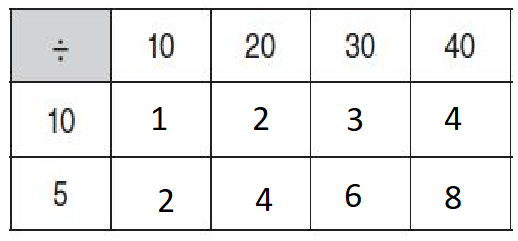Question 16.
Part B
Find the quotient, a.
80 ÷ 10 = a
a = ______

Explanation:
80 ÷ 10 = 8.
The quotient is 8.

How could you use a to find the value of n?
Find the value of n.
80 ÷ 5 = n
n = ______
a = ______
n = ______

a= 8.
n= 16.

Explanation: By doubling the value of ‘a’ we can get the value of ‘n’. As the value of ‘a’ is 8, so the value of ‘n’= 8+8= 16

Question 17.
Eve needs 2 limes to make a glass of limeade. If limes come in bags of 12, how many glasses of limeade can she make using one bag?
______ glasses

Explanation: The limes in the bags are 12 and Eve needs 2 glasses of limeade, so the number of glasses she can make is 12÷2= 6 glasses.

### Test – Page 6 – Page No. 76

Question 18.
For numbers 18a–18e, select True or False for each equation.
a. 18 ÷ 9 = 2
i. True
ii. False

Explanation: As 18 ÷ 9 = 2, so the answer is true.

Question 18.
b. 27 ÷ 9 = 4
i. True
ii. False

Explanation: As 27 ÷ 9 = 3, so the answer is false.

Question 18.
c. 45 ÷ 9 = 5
i. True
ii. False

Explanation: As 45 ÷ 9 = 5, so the answer is true.

Question 18.
d. 72 ÷ 9 = 7
i. True
ii. False

Explanation: As 72 ÷ 9 = 8, so the answer is false.

Question 18.
e. 81 ÷ 9 = 8
i. True
ii. False

Explanation: As 81 ÷ 9 = 9, so the answer is true.

Question 19.
Holly is making 4 vegetable trays for a party. She wants to divide 36 carrot sticks equally among the trays. How many carrot sticks will she put on each tray?
______ carrot sticks

Explanation: No of carrot sticks on each tray = (Total carrot sticks ÷ no of vegetable trays)
= 36 ÷ 4
= 9 carrot sticks in each tray

Question 20.
Hector is buying books at a book store.
Part A
He buys 2 used books and 1 new book for $26. The new book costs$18. Each used book costs the same amount. What is the price of each used book? Explain the steps you used to solve the problem.
$______ Answer: Each used book costs$ 4.

Explanation:
Given that cost of one new book = $18 Cost of 2 used books + 1 new book =$ 26
Cost of 2 used books = 26 – 18 = 8
Cost of 1 used book = 8/2 = $4 Question 20. Part B Hector also buys a reading light for$12 and 2 journals for $8 each to give as gifts. Write one equation to describe the total amount Hector spends on gifts. Explain how to use the order of operations to solve the equation. Total amount:$ ______

Answer: $28. Explanation: Hector bought a reading light for$12
2 journals for $8 each Total money spent on gifts = 12 + (2 x 8) = 12 + 16 =$ 28.

Conclusion:

This assessment test helps students to check their math skills. Go Math Grade 3 Chapter 10 questions are explained in detail that students can understand easily.

Scroll to Top